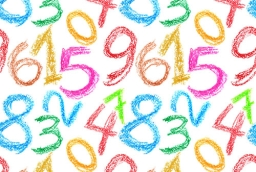# 4 digit number

I am a four-digit number. My thousand period has a first digit which is thrice the second digit, and the second digit is two more than the third digit. All the rest of the digits are zeros. What number am I?

x1 =  6200
x2 =  9310

### Step-by-step explanation:Did you find an error or inaccuracy? Feel free to write us. Thank you!

Tips for related online calculators
Do you have a linear equation or system of equations and looking for its solution? Or do you have a quadratic equation?